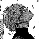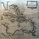1029 visningar
Coders,

I am working on this adaptive SMA . It has its pros and cons. It is a work in progress and I welcome any one that wants to add or change it. If you add or make positive changes please let me know. It is based off of daily range. Currently it is set to the open three days prior to the current open.
```//@version=2
length = input(title="SMA Length",defval=200)
f=input(title="range factor",defval=16)
rs=input(title="range smoother",defval=100)
r=abs(open-open)*2000
ra=round(sma(r,rs))
l=iff(ra*f>length,length,length-ra*f)
sum = 0
for i = 0 to (l-1)
sum := sum + close[i]

plot(sma(close,length),color=yellow,linewidth=3,transp=0)

//l=len+aa*f
//    f*aa
//else
//    for i = 0 to 60
//            f*aa
//            break
//        else
//            continue
//a=sma(close,34)
//b=round((abs(close-a))*200)
//c=iff(b*f>=len,len,len-b*f)```

## KommentarerHi friend. I don't know if you're still active but I just want to let you know this is one of the best Adaptive Moving Averages I have ever seen. Is it possibly to add multiple adaptive moving averages to the script? Or add a specific period as in, I want to see the adaptive moving average of the 15 min chart on the 5 min chart. Is any of this possible? Thanks.
SvaraBenevolentStockCharts
@BenevolentStockCharts, Hi Edwardv, thanks for the compliment on the code I wrote. It feels like many years ago that I wrote it. I made another based off of standard deviation years back. Multiple adaptive averages can be thrown on the plot. Just duplicate the code, change variable names.
Svaraforexpirate
@forexpirate, Thanks for the reply! I'm not a good coder but I'll try. Again, thanks for creating this wonderful script!
SvaraBenevolentStockCharts
@BenevolentStockCharts, I can give it a go. I find technical analysis to be largely voodoo; however, I too get sucked into the search for the 'idea', the collection of equations that will produce tradeable signals.
Svaraforexpirate
@forexpirate, If you have time to write up the script to allow multiple ASMA that would be awesome man. Genuinely a great script. I've been using ASMA 50 and ASMA 74 on the 105 minute chart and it's a blast!
SvaraBenevolentStockCharts
@BenevolentStockCharts, //@version=2
length = input(title="SMA Length red",defval=81)
length2 = input(title="SMA Length yellow",defval=144)
length3 = input(title="SMA Length lime",defval=250)
f=input(title="range factor",defval=16)
rs=input(title="range smoother",defval=100)

//f2=input(title="range factor",defval=16)
//rs2=input(title="range smoother",defval=100)

r=abs(open-open)*8
ra=round(sma(r,rs))
l=iff(ra*f>length,length,length-ra*f)
sum = 0
for i = 0 to (l-1)
sum := sum + close

//r=abs(open-open)*8
//ra=round(sma(r,rs))
l2=iff(ra*f>length2,length2,length2-ra*f)
sum2 = 0
for i = 0 to (l2-1)
sum2 := sum2 + close

//r=abs(open-open)*8
//ra=round(sma(r,rs))
l3=iff(ra*f>length3,length3,length3-ra*f)
sum3 = 0
for i = 0 to (l3-1)
sum3 := sum3 + close

Svaraforexpirate
@forexpirate, It has been a while since I wrote that. I pump out code and I don't name variables well. So, when I look back I wonder what the hell I meant. This is not the one based off of standard deviation. Maybe that one is better; maybe not. There are three inputs, put in your SMA values and play with it. What do you use this on? Stock, FOREX, or bitcoin types.
Svaraforexpirate
@forexpirate, length = input(title="SMA Length red",defval=50)
length2 = input(title="SMA Length yellow",defval=74)
length3 = input(title="SMA Length lime",defval=105)

Change defval to your numbers, works better long term if you make changes. It will always default to your values.
Svaraforexpirate
@forexpirate, Whoa thanks man. I'm pasting the code onto the pine editor and getting an error in line 18. I've been using 20/50/74 on Crypto and Stocks and it's been REALLY great. Haven't tried it on Forex yet.
SvaraBenevolentStockCharts
@BenevolentStockCharts, Try changing the SMA values one number, the code does get sensitive

Svara